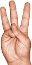# Mental Calendar Math

### Find the Day of the Week for Any Date

To find the weekday for a date we add the day number, a month code, and a year code, working in "weekday arithmetic" (wrapping back to zero when we hit seven). You have to memorize the month codes and calculate the year code, so it gets a little complicated, but we'll take it in stages.

First we number the days: I've chosen (somewhat arbitrarily) to start with Sunday as 0.

1. Sunday ("Noneday")
2. Monday ("Oneday")
3. Tuesday ("Twosday")
4. Wednesday ("ednesday")
5. Thursday ("Foursday")
6. Friday ("Fiveday")
7. Saturday ("Sixturday")

Armed with the information that YYYY is a “y” year, while MM is a “m” month, you can now practice finding the weekday for dates in the current month. Add the year and month codes to the day number, then throw out sevens to get the day of the week (divide by seven and take the remainder, or just subtract seven repeatedly).

Choose the correct day below:

MM DD, YYYY is a: Sun Mon Tues Wed Thurs Fri Sat

Now for the memorization part. The month code gives the day before the first of the month (because we're going to add 1 to get the “first” day). So although you might expect January's code to be 0, it is actually 6.

In a leap year, there is an extra day at the end of February. We pretend this extra day is at the very beginning of the year (by including it as part of the year code). But then we need to subtract 1 from January and February's codes (because the extra day hasn't happened yet).

Since they're all single digits, I like to group the month codes into four 3-digit numbers for memorization. Note the similarity between the first and fourth quarters, and between the second and third.

Jan/Feb/MarApr/May/June July/Aug/SepOct/Nov/Dec
6, 2, 25, 0, 3 5, 1, 46, 2, 4

But if you prefer them grouped by day:

May Sunday (0) Monday (1) Tuesday (2) Wednesday (3) Thursday (4) Friday (5) Saturday (6)

Now you can find the weekday of any date in the current year. But we'll choose day (or a ) so you can concentrate on the month codes:

MM DD, YYYY is a: Sun Mon Tues Wed Thurs Fri Sat

The year code is the weekday of the first day (January 1st). Calculating it is more involved, so you could stop here. You get most of the practical value of this technique by remembering the current year code. But if you want to do the party trick where you tell people the day of the week for arbitrary dates...

A year is 365 days. That's 52 weeks and one day, so each normal year starts one weekday later than the previous year. Then every fourth year is a leap year which adds another day.

So the year code is the year plus the number of leap days (the year divided by four). To make it possible to work this in your head, we can (mostly) use a two-digit year. So in 2016 we add 4 leap days (16/4): 16 plus 4 is 20. Convert that to a day number and you get 6.

While it's perfectly possible to do that calculation directly, it can be a bit intimidating, especially toward the end of a century. And since all we need is the weekday, there's a trick which lets us do (more) easier operations on smaller numbers.

Instead of doing the division by four, just find the next smaller multiple of four and the remainder. To do this, look at the tens digit. If it's even, then multiples of four have a ones digit of 8, 4, or 0. If it's odd, multiples of four have a ones digit of 6 or 2.

Remember the remainder and divide the other number in half, then find the distance to the next multiple of 7.

Add this distance to the remainder to get the year code.

Take year (or a ):

 YY = 4q + r. 4q / 2 = 2q. ?? = 2q + s. r + s = year code. vs. YY / 4 = q. YY + q = yc. yc % 7 = year code.

Now you can find the weekday of any date this century (20XX).

But...a century doesn't come out to an even number of weeks. And our calendar has some fine-tuning corrections: three out of four centuries (years ending in 00) are not leap years. So using a two-digit year isn't quite right: we also need to apply a century correction. We take the remainder from dividing the century by four, and subtract twice that from the year code.

But you don't have to calculate this: you can just remember it. The Gregorian calendar was invented in 1582 and wasn't adopted by the British Empire until 1752, so there aren't many centuries to worry about. Note that we can express these all as positive numbers since (for instance) subtracting 6 days gives the same weekday as adding 1.

 20xx +0 +1 +3 +5 +0 +1

Now that's really all, so here's a widget that will let you drill with random dates.

 to Jan Feb Mar Apr May June July Aug Sep Oct Nov Dec to Jan Feb Mar Apr May June July Aug Sep Oct Nov Dec 1 2 3 4 5 6 7 8 9 10 11 12 13 14 15 16 17 18 19 20 21 22 23 24 25 26 27 28 29 30 31 to 1 2 3 4 5 6 7 8 9 10 11 12 13 14 15 16 17 18 19 20 21 22 23 24 25 26 27 28 29 30 31

MM DD, YYYY is a: Sun Mon Tues Wed Thurs Fri Sat